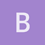This is an archived static version of the original phylobabble.org discussion site.

# Graphing identical sequences on phylogenetic tree using R and APEblarsen_brendan

Hello I have phylogenetic trees of deep sequencing data. The problem is that many of the sequences are identical so i wanted to have those nodes that have x number of identical sequences be represented by a circle of size x. This is easily enough done in R using the APE package. The problem is I have two groups of sequences that I want to color differently. So at node 1 for example, 30% of the identical sequences come from group 1, and 70% come from group 2. Ideally these circles at nodes would actually be pie charts that show the different representation but I cannot figure out how to calculate the vector to feed ape. any ideas?

Thanks, Brendanboronian

Hi @blarsen_brendan, maybe I miss part of your problem, but this should be possible with phyloch::descendants (http://www.christophheibl.de/Rpackages.html) to get the percentages and ape::nodelabels to plot the pie charts.

Does that help?boronian

Does this what you want (In the random tree the clades might be nested in each other, but I assume you have the list of nodes for which this operation would be needed)?

require(phyloch)
r.tre<-rtree(10)
nodes<-c(19,16,13)
ga<-c("t5","t9","t2")
gb<-c("t1","t3","t4","t6","t7","t8","t10")
perc.a<-c()
perc.b<-c()
for (i in 1:length(nodes))
{
leaves<-descendants(r.tre,nodes[i], labels=T,type = "t")
sharea<-length(which(leaves%in%ga))
shareb<-length(which(leaves%in%gb))
perc.a[i]<-sharea/(sharea+shareb)
perc.b[i]<-shareb/(sharea+shareb)
}
pietab<-cbind(perc.a,perc.b)
plot(r.tre)
nodelabels(,nodes,pie=pietab)


Cheers